# 1N4148 Reverse Bias Leakage Current

All semi-conductor diodes have some amount of reverse-bias conductance. In the case of a 1N4148, reverse leakage current is guaranteed to be less than 25 nA when reverse-biased at -20 VDC at room temperature (25 degC). In this post we will measure the reverse biased I-V curve of 4 1N4148 diodes.

The standard spice model of a diode under reverse bias is the following,
$I_D = \begin{cases} I_s\left(e^{\eta qV_D/kT} -1 \right) + V_D\text{GMIN} &\quad\text{for} & -5\dfrac{\eta kT}{q} \leq V_D \leq 0 \\ \\ -I_s + V_D\text{GMIN} &\quad \text{for} & V_D < -5\dfrac{\eta kT}{q}\\ \end{cases}$
When the DUT is reverse biased from 0 mV to -250 mV, we expected to observe a large rise in leakage current. For reverse bias voltages less than -250 mV, we expect to see a constant current source with a high parallel resistance.

## Measurement Setup

Various spice models provide the saturation current of a 1N4148 to be around 2 nA. Anticipating the leakage current will be on the order of 1 nA to 10 nA, we can choose a suitable current measurement technique. The minimum current measurement range of the 34401A multimeter is 10 mA. At 5-1/2 digit resolution (10 PLC) the current resolution 10 nA. At 6-1/2 digit resolution (100 PLC) the resolution is 1 nA. Neither of these modes are particularly attractive for a signal which is maximally 10 nA. If the 34401 was employed in DCI mode, a total of 10 ADC counts would be available.

Alternatively, we can use a current shunt resistor and measure the voltage developed across the current shunt resistor. Fortunately, the 34401A has the ability to provide a high input-resistance on its input terminals (Hi-Z mode). A schematic of the test setup is shown below,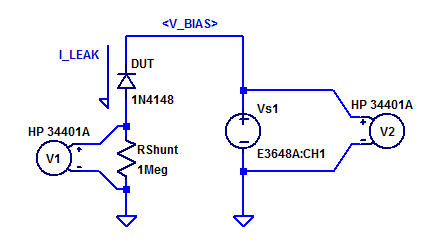Here a 1 MOhm resistor is used as a current shunt resistor. Voltmeter V1 samples the potential across $$R_{shunt}$$. Voltmeter V2 samples the bias potential being applied to the DUT. Power supply Vs1 is stepped programmaticly from 0 VDC to -20 VDC.

While a breadboard isn’t the ideal choice for low-level measurements, it is convenient for testing through-hole components. Test-jig leakages is assessed by applying a DC bias of 20 VDC to various nodes on the breadboard and recording shunt voltage. This leakage test, resulted in maximally 10 pA of leakage to the shunt resistor. For a first pass look at a 1N4148 with 1 nA of leakage, it will serve well for this blog post.

A photo of one DUT in the test setup is shown below.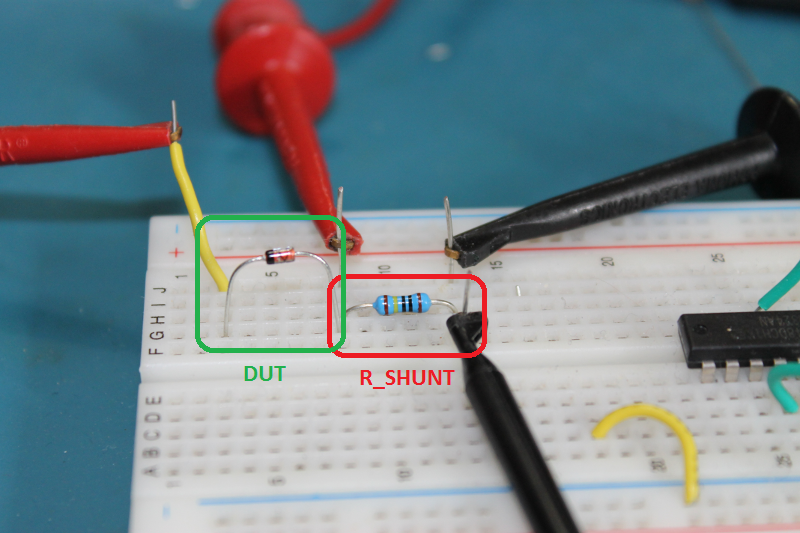## Measurement Results

Room temperature and the shunt resistor are recorded as,

$$\begin{matrix} T_{amb}\; : & 22.6 \; ^oC \\ R_{Shunt}\;: & 1.0009 \; M\Omega \end{matrix}$$

Each of the 4 1N4148 were reverse biased from 0 to – 20 VDC. The resulting leakage current can be seen in the figure below.A figure with a reduced span of reverse bias voltages greater than – 250 mV is shown in the figure below.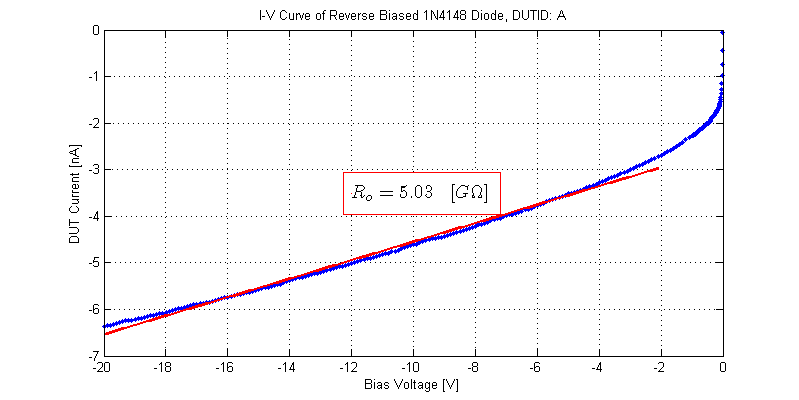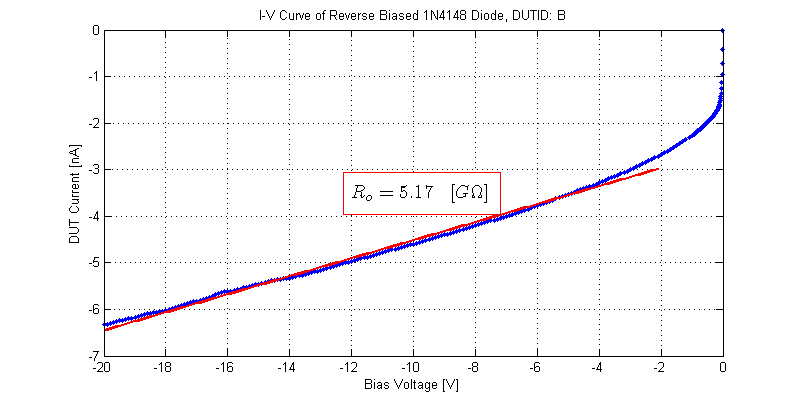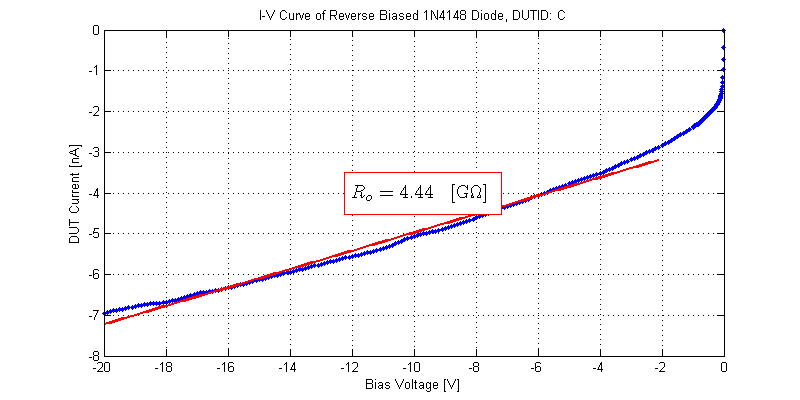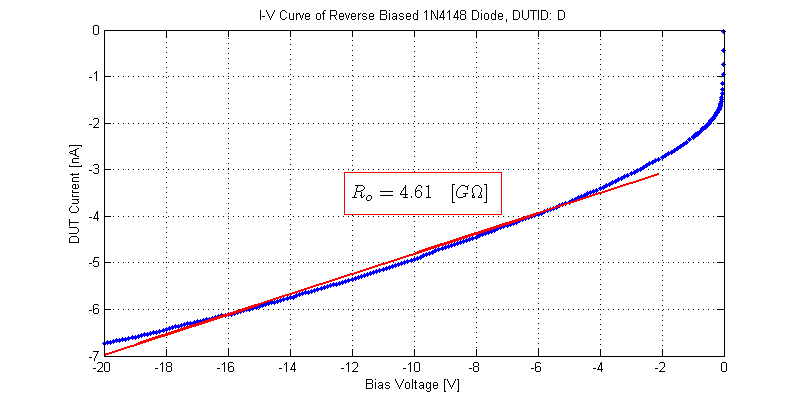The blue excursion at approximately -1 VDC, is the 34401 range switching on the volts DC measurement of DUT reverse bias potential. Longer integration periods when measuring Rshunt would produce a cleaner plot (at the expense of test time).

By far the largest challenge in modelling these 4 sample 1N4148 diodes is the non-linear reverse bias conductance. Contrary to the spice model introduced above, these DUTs never reach a region of constant conductance. The piece-wise model defines the region of constant conductance as $$V_D$$ < $$-5\eta V_T$$. Assuming $$\eta \approx 2$$, for reverse bias potentials less than -250 mV, the conductance should be constant. Even worse is that GMIN (conductance) is a global spice parameter not a unique component attribute. With GMIN having a typical default value of GMIN = $$10^{-12}$$ mho, which is equivalently a shunt resistance of 1 TOhms.

## Aside

Even the typical response described in the datasheet is not observed on these 4 sample DUTs. A graph showing the typical reverse bias leakage taken from the following datasheet is shown below.## Raw Data

The I-V curves collected for the 4 DUTs can be found as csv files below:

Files are formatted as,
DUT Voltage [V], DUT Current [A]

## 1 thought on “1N4148 Reverse Bias Leakage Current”

1.Vince Patron says:

Thanks for publishing your data! Doing a 2 minute timer/oscillator circuit with a small ceramic 4.7uF cap so needed a diode with very low leakage. Looks like the standard 1N4148 will work fine.

This site uses Akismet to reduce spam. Learn how your comment data is processed.